Home / Math Worksheets / Kindergarten Math Worksheets

# Free Printable Kindergarten Math Worksheets PDF

Our free Kindergarten Math Worksheets in PDF were created for you to use at home or with kids in the classroom. Download printable math worksheet for kindergarten which teach basics of addition, subtraction, shapes recognition, comparing numbers and much more.

Try also our Math Games for Kindergarten here →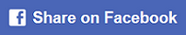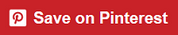### Missing numbers

Missing numbers for Kindergarten 1-10.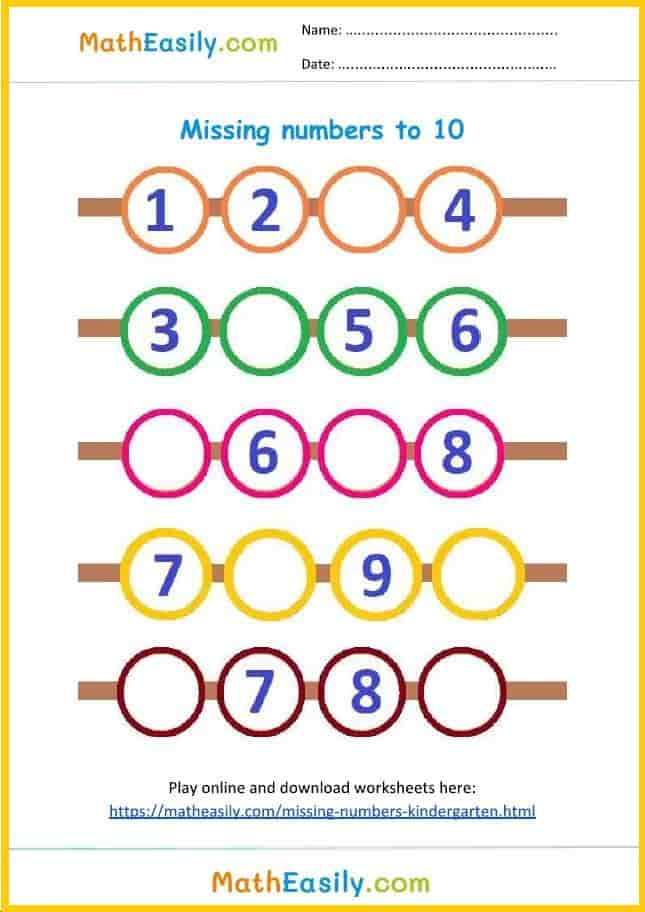### Missing numbers

Missing numbers for Kindergarten 1-10.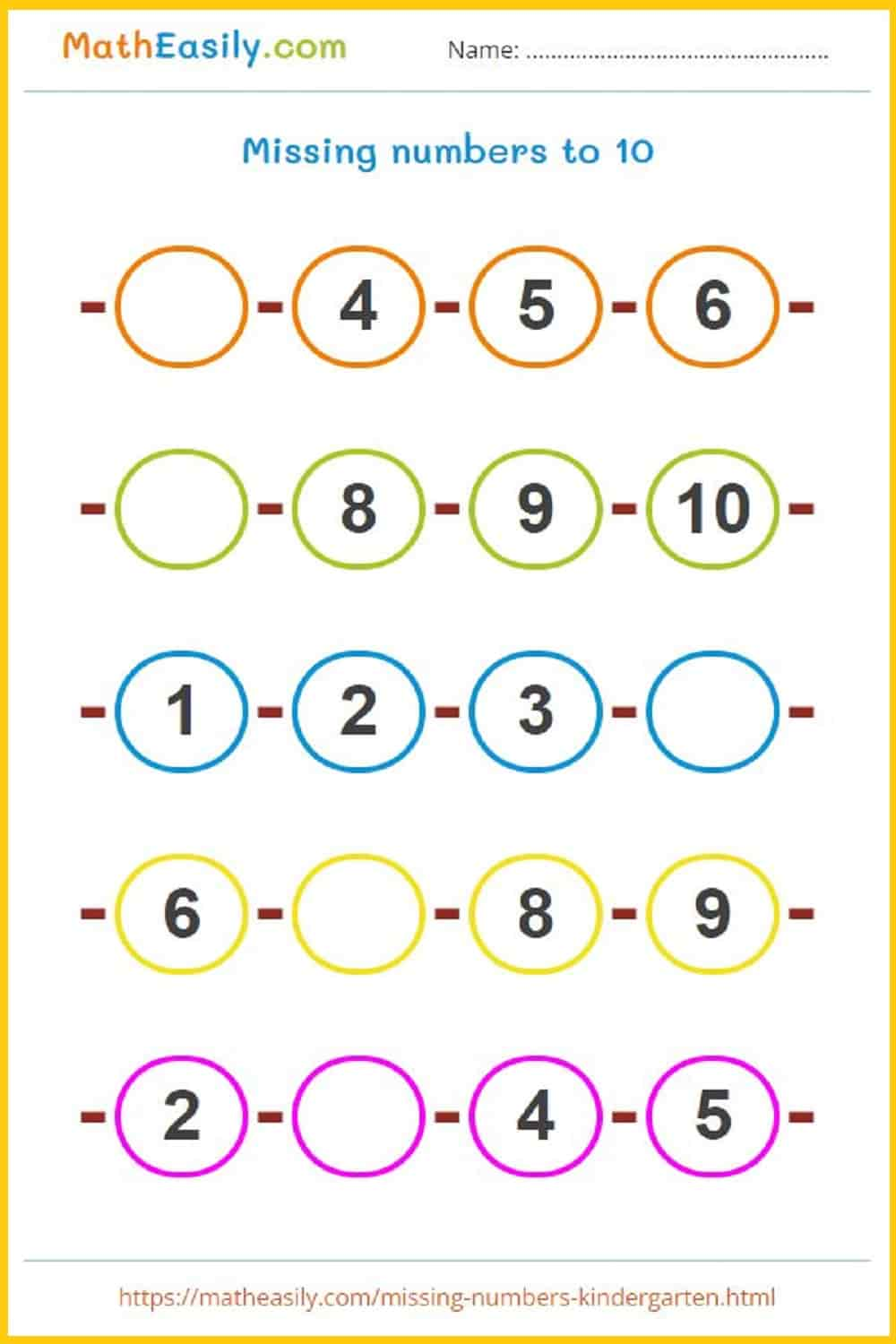### Missing numbers

Missing numbers for Kindergarten 1-10.### Counting games

Counting games for kindergarten.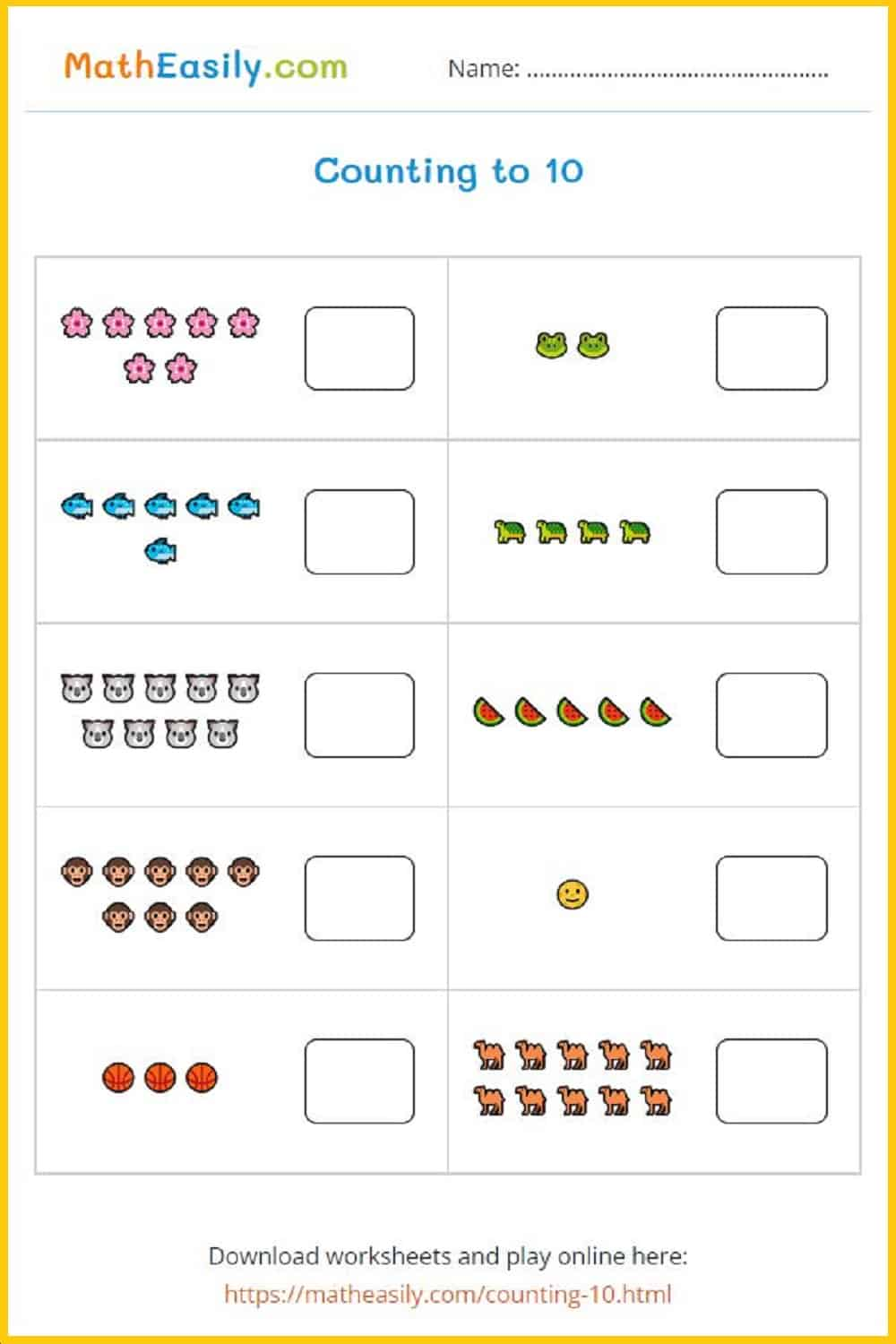### Counting to 10

Count objects and write the number 1-10.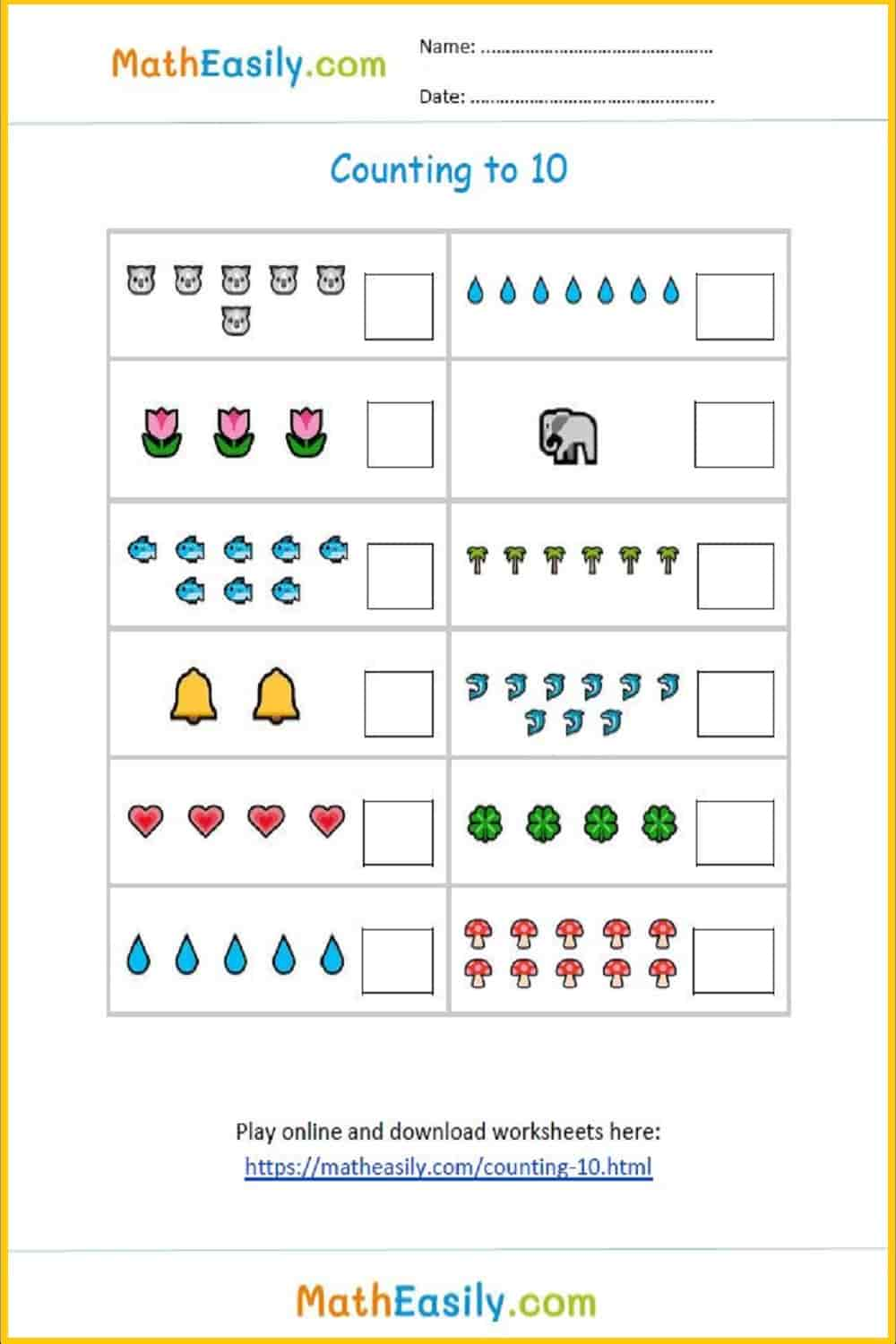### Counting game

Count objects and write the number 1-10.### Compare numbers

Comparing numbers for Kindergarten. Select the correct symbol.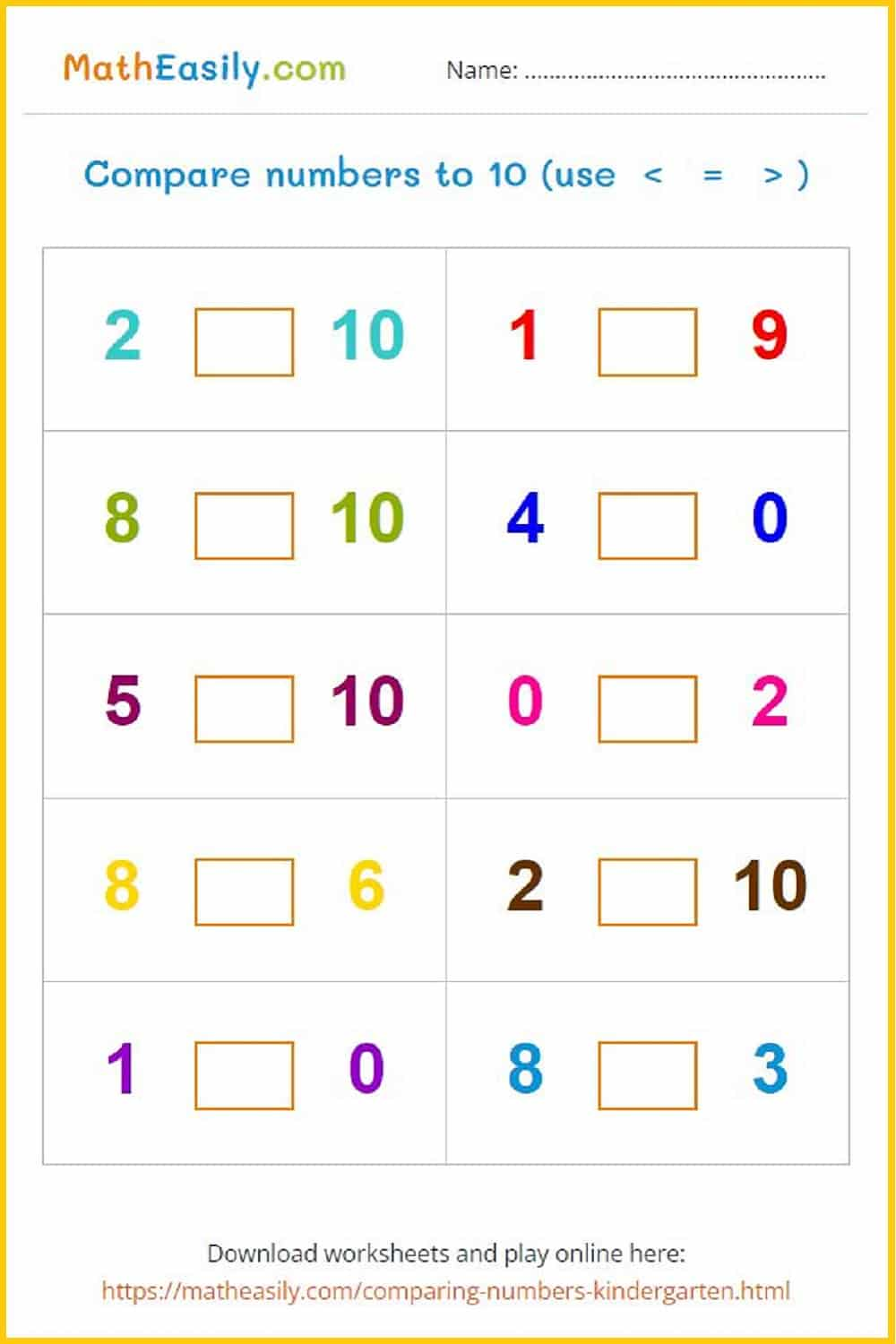### Compare numbers

Comparing numbers for Kindergarten. Select the correct symbol.### Compare numbers

Comparing numbers for Kindergarten. Select the correct symbol.### 2D Shapes Chart### Identify Shapes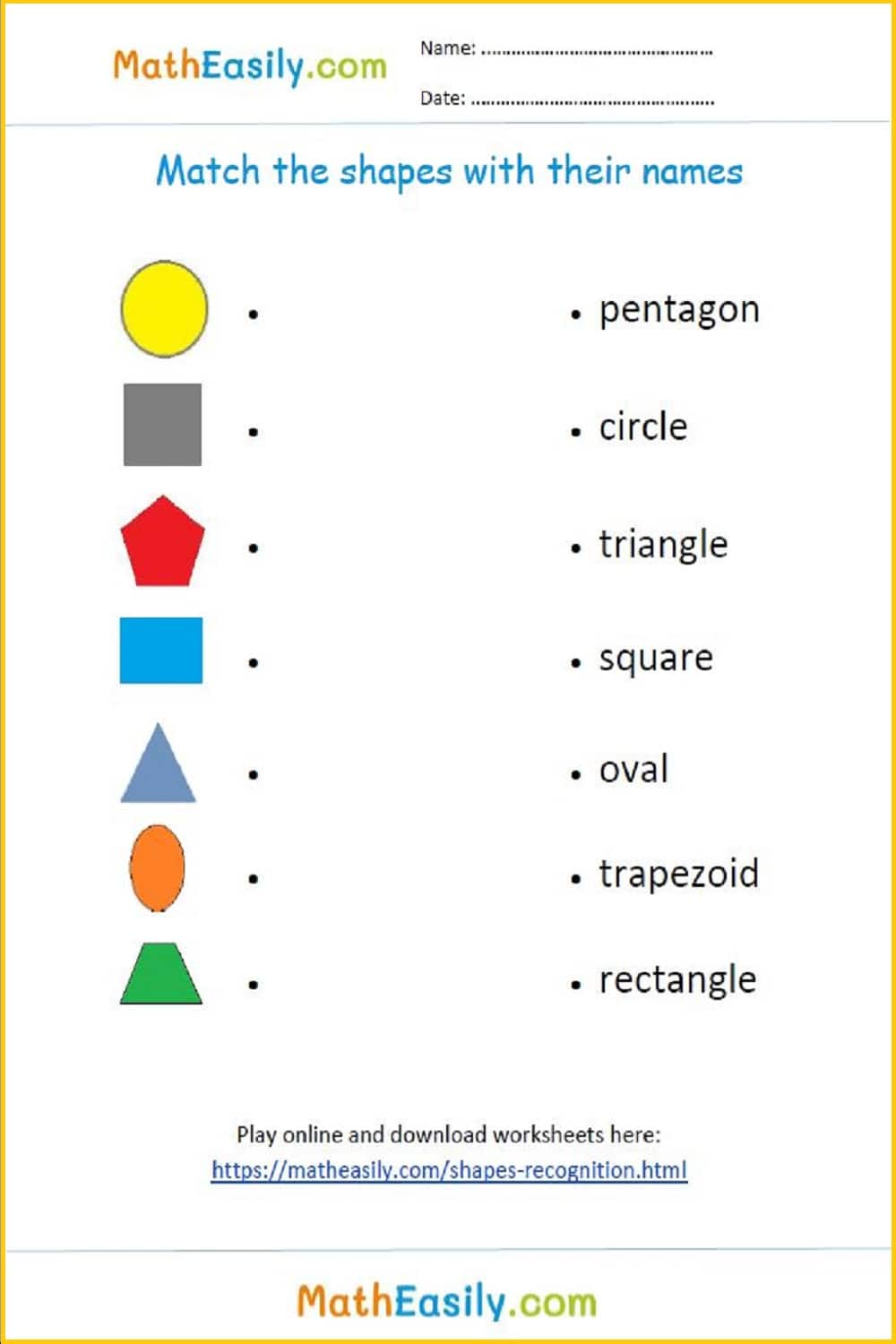### Shapes Recognition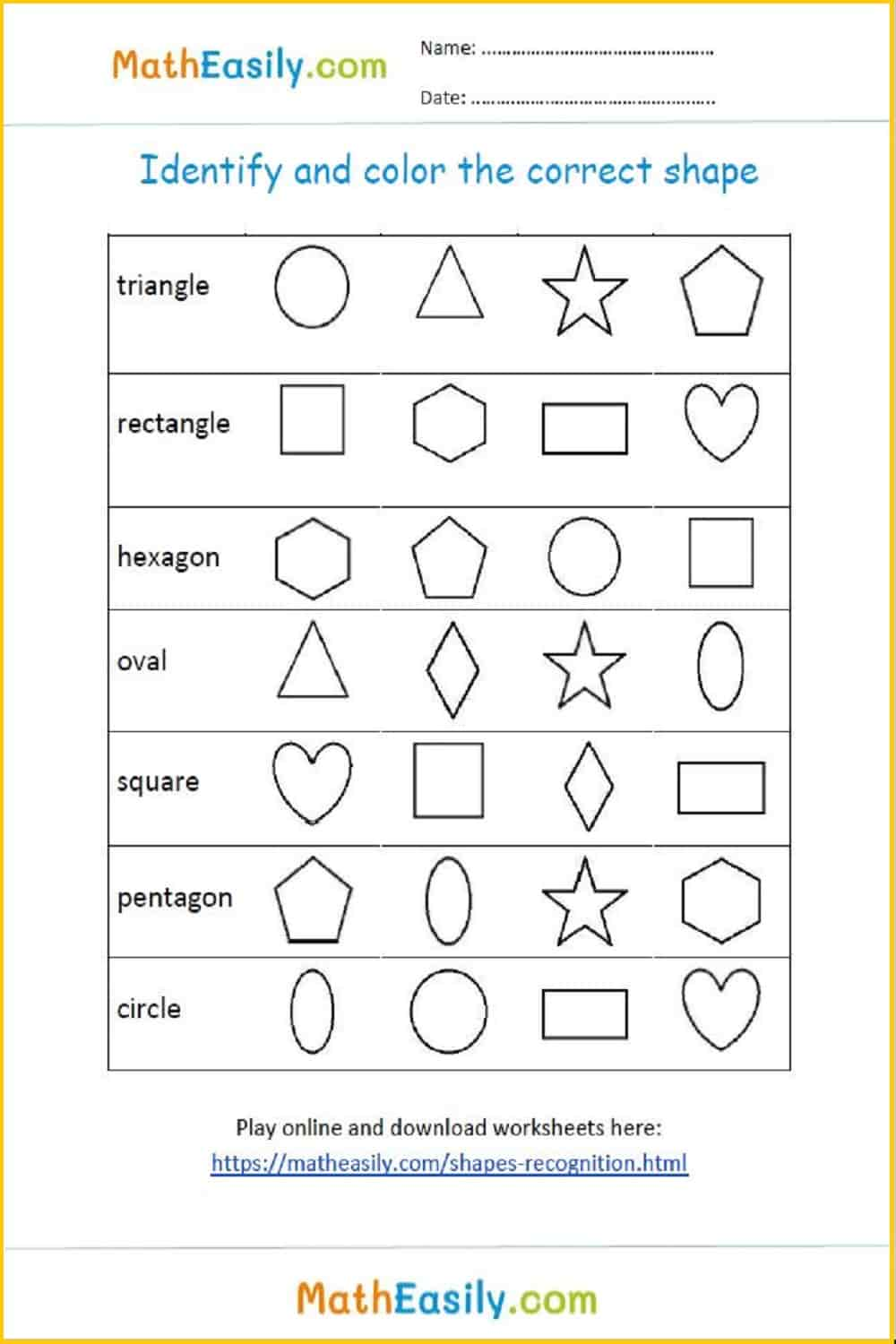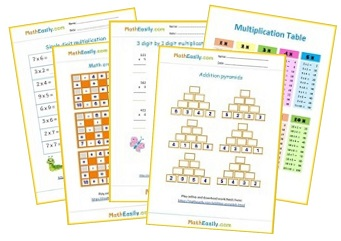Free Math Worksheets

## Math Worksheets for Kindergarten ADDITION

Dice game: single digit addition up to 20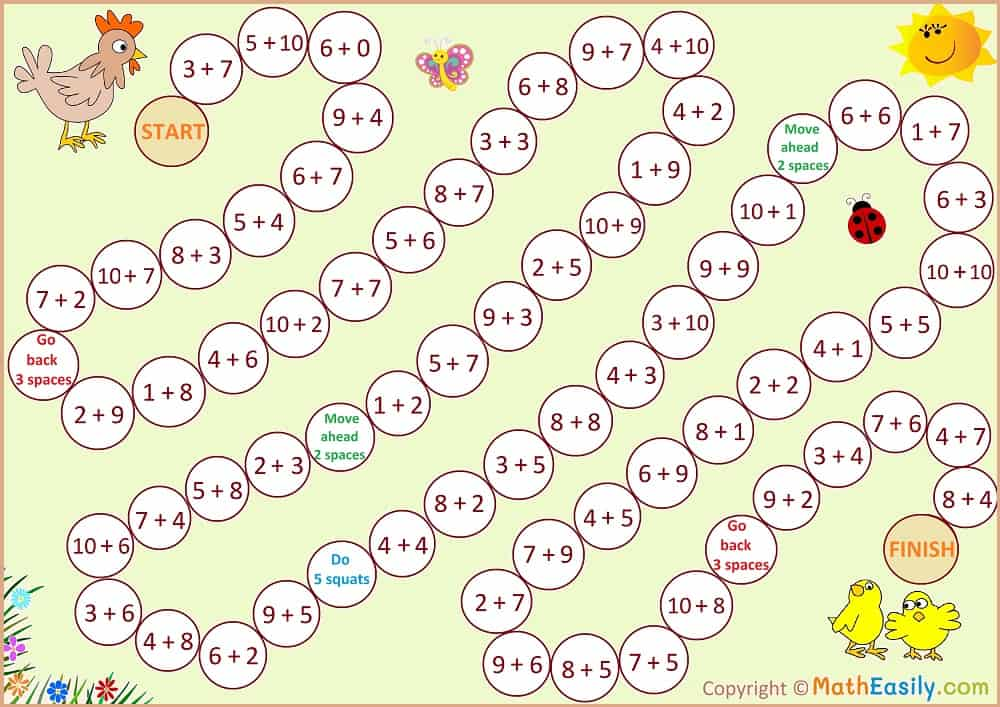Addition up to 10 with help of pictures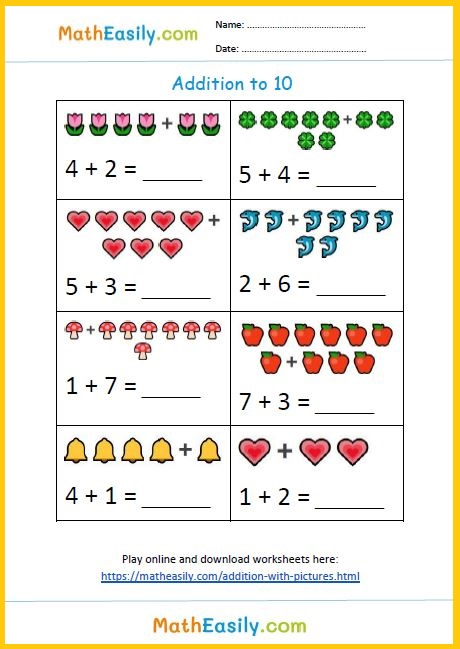Dice game: single digit addition up to 12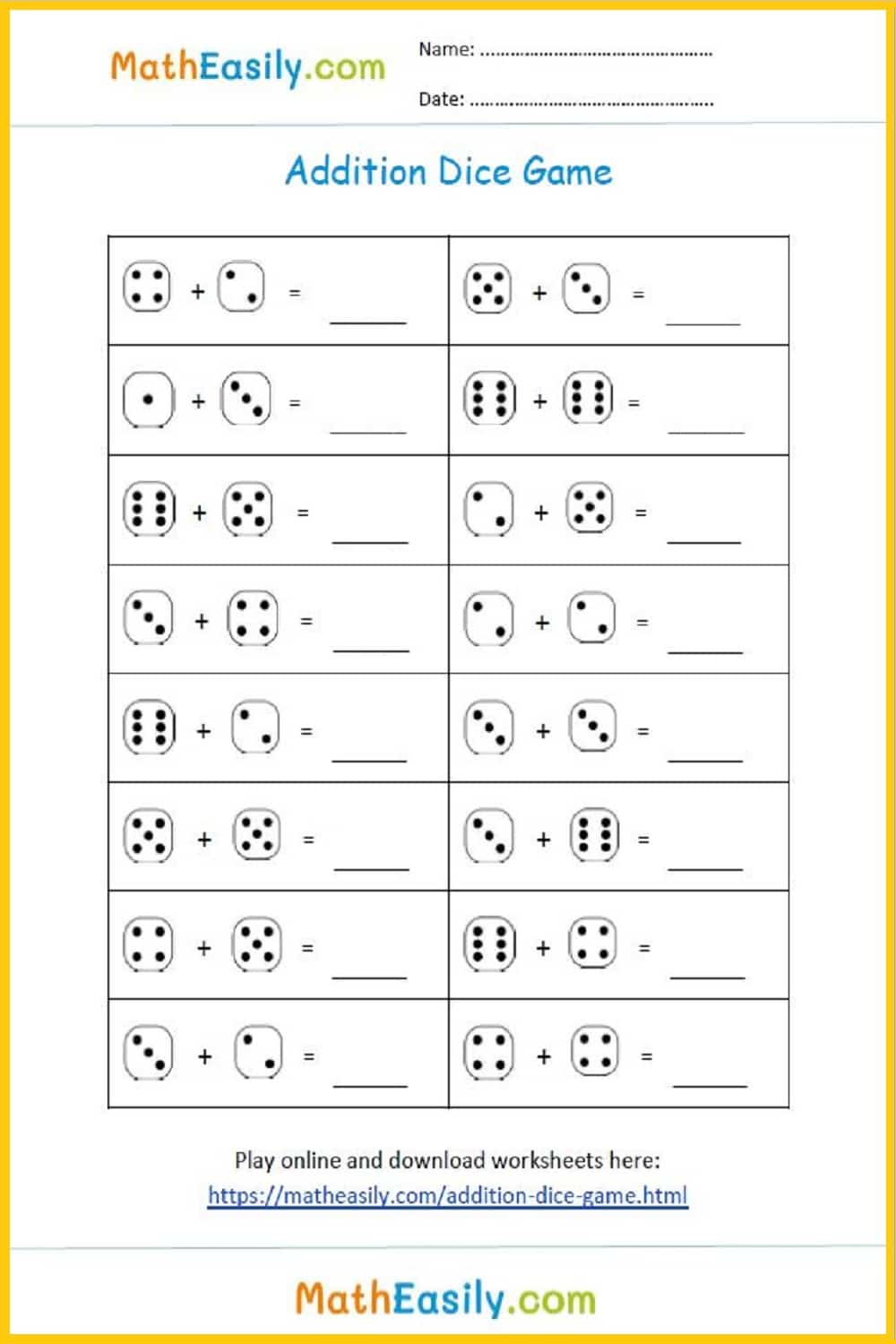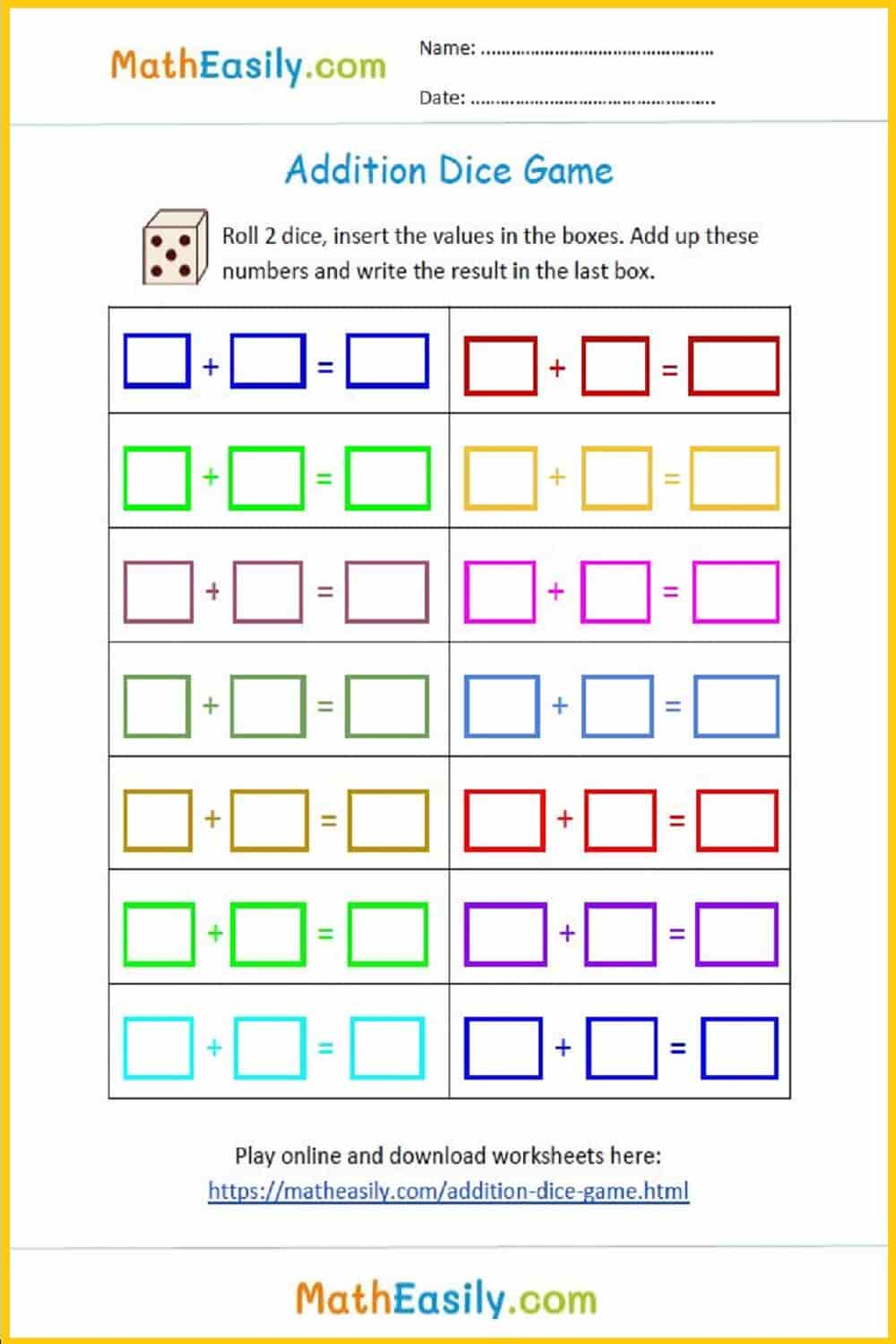Addition up to 20 with help of picturesFill in the addition pyramid puzzles up to 30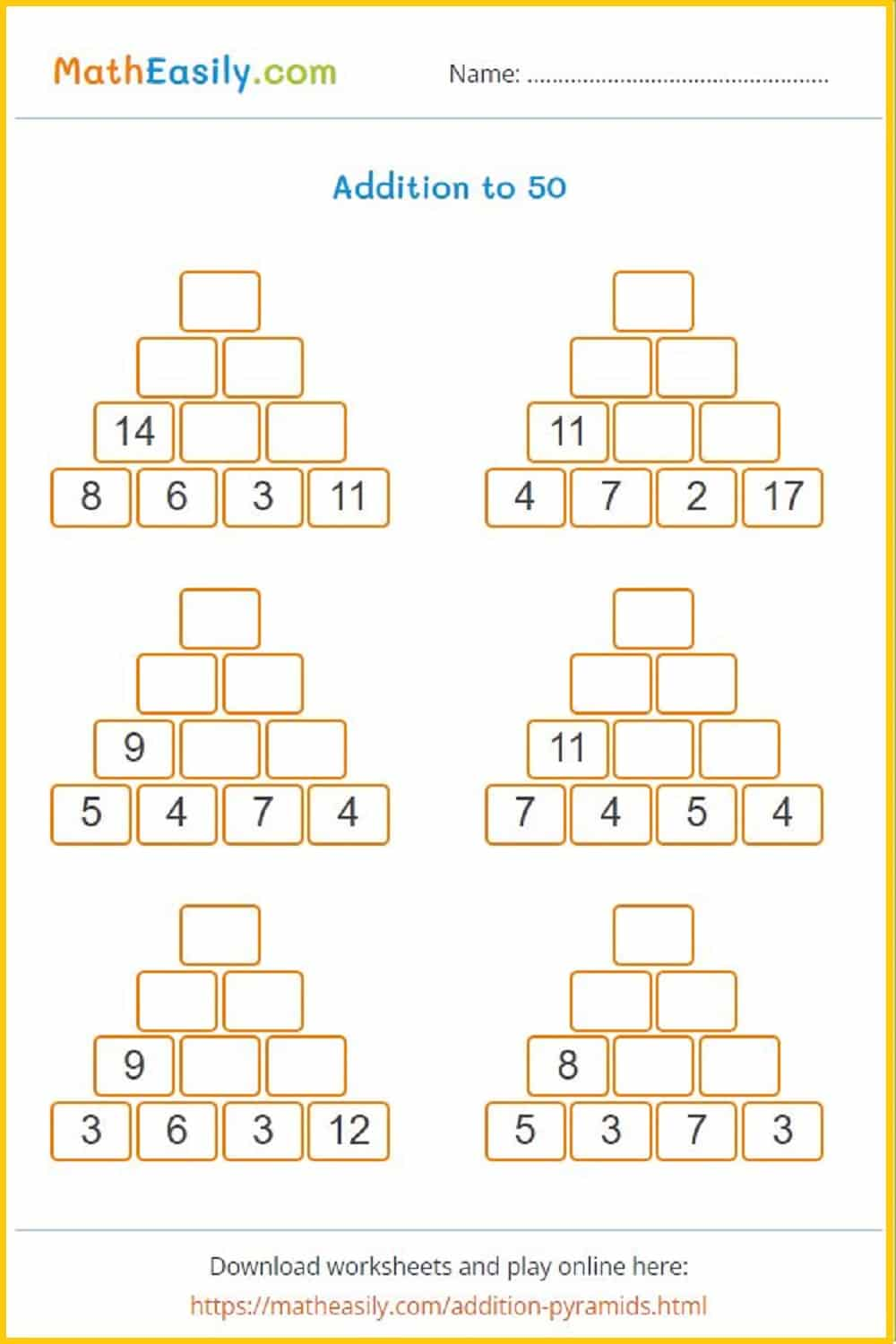Fill in the addition pyramid puzzles up to 30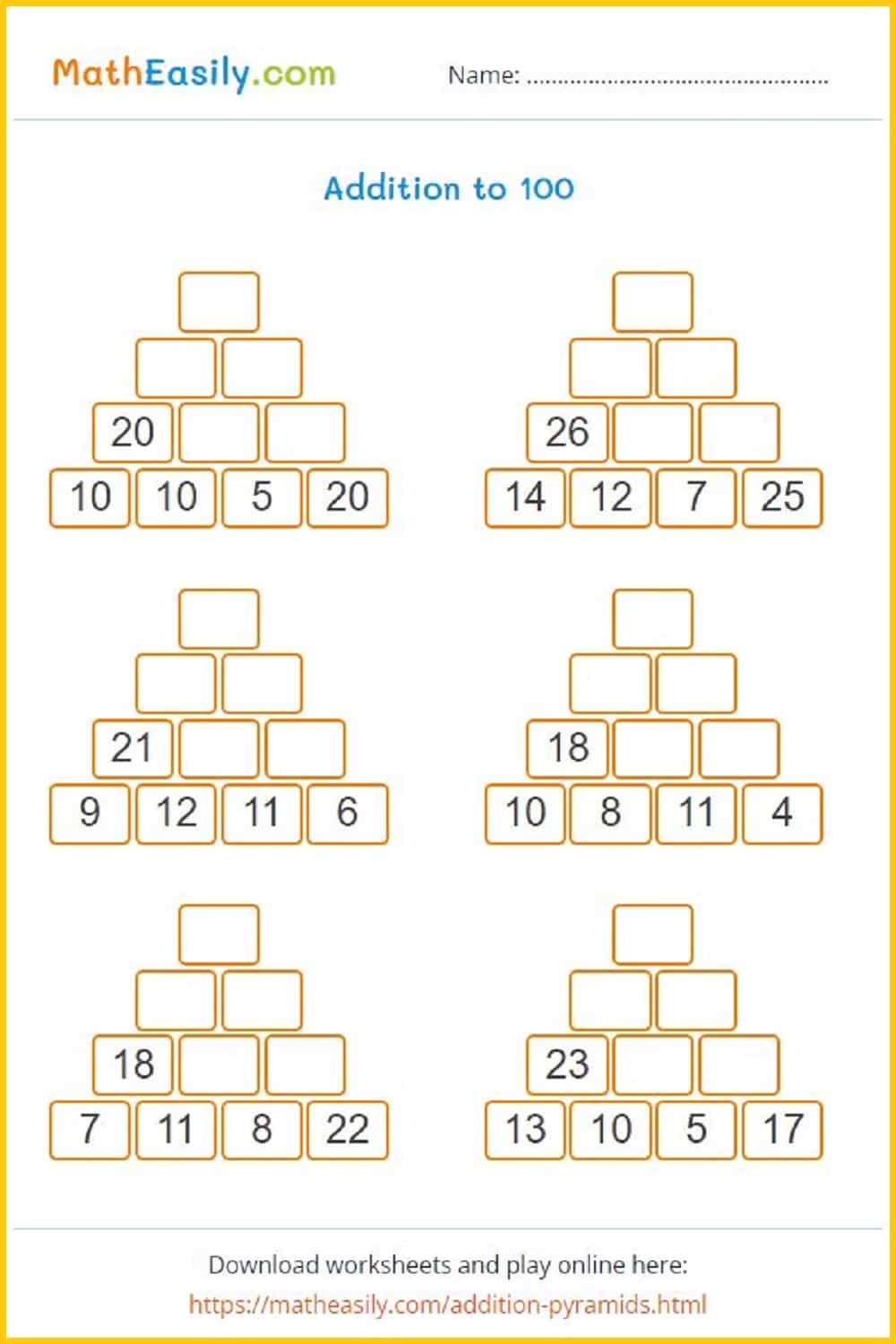Fill in the addition pyramid puzzles up to 30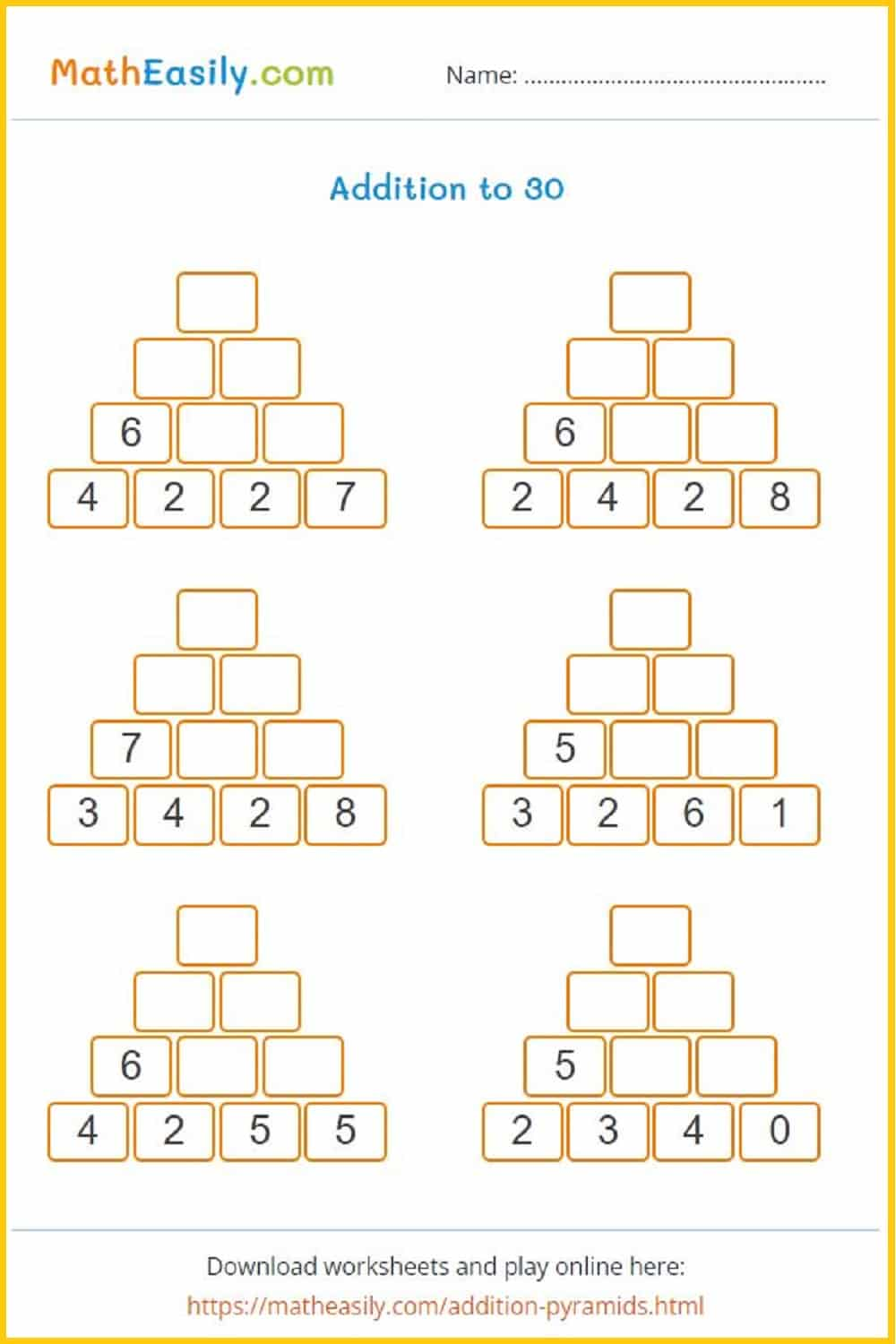## Free Math Worksheets for Kindergarten SUBTRACTION

Download printable math worksheets for kindergarten (subtraction). These subtraction worksheets for kindergarten teach kids the basics of subtraction.

### Single digit subtraction

Subtraction within 10 with help of pictures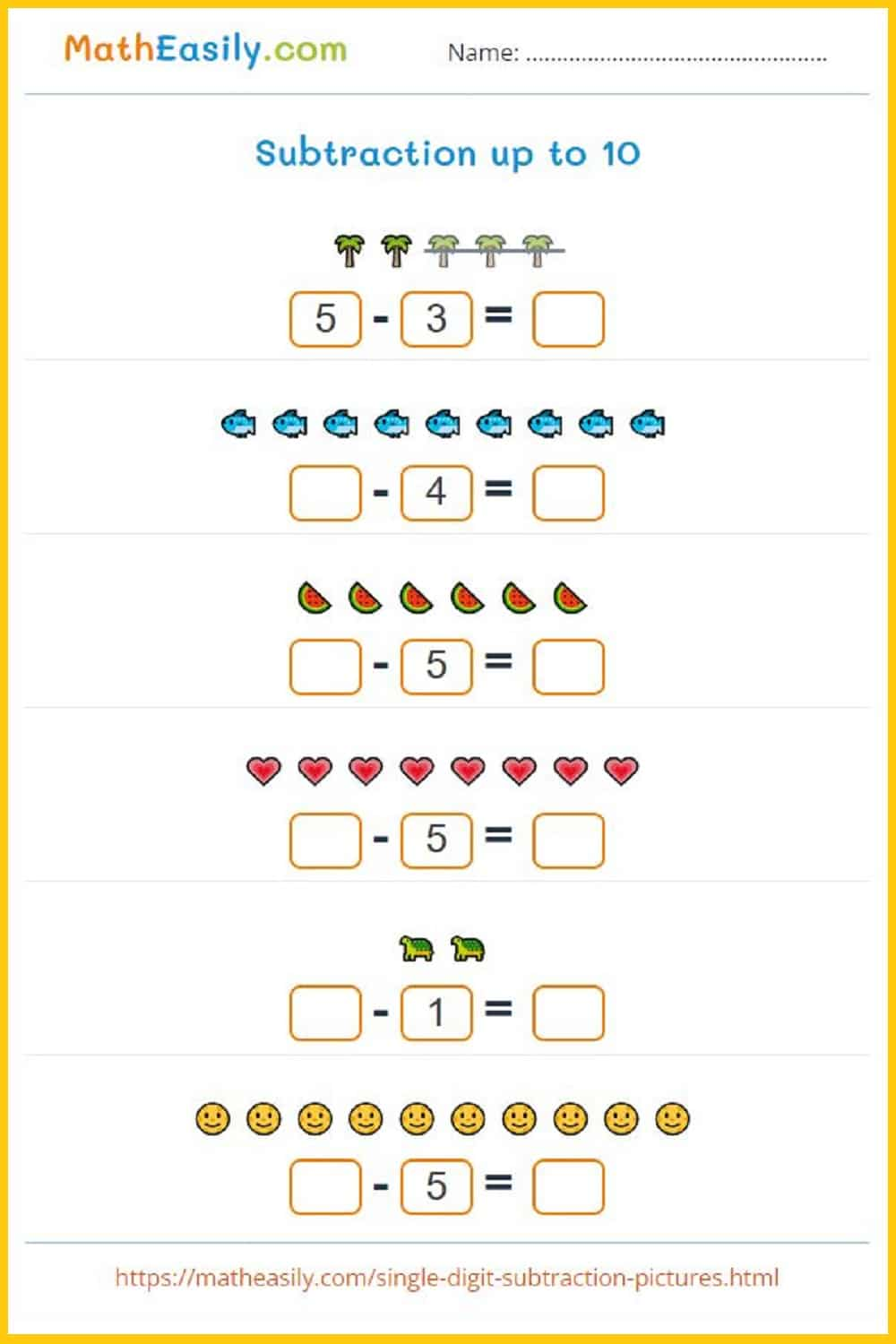### Subtraction within 10

Single digit subtraction with help of pictures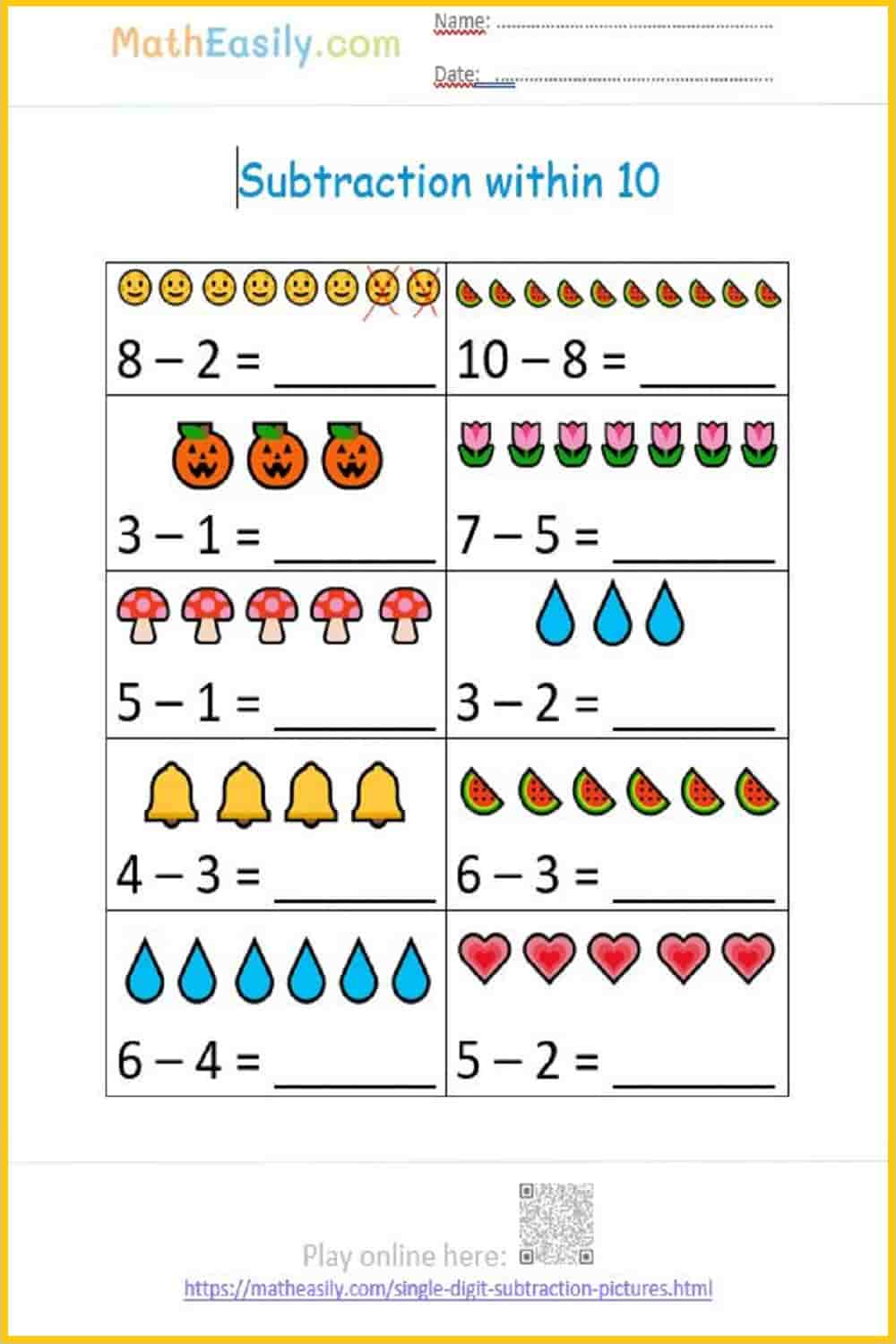### Single digit subtraction

Subtraction within 10 with help of pictures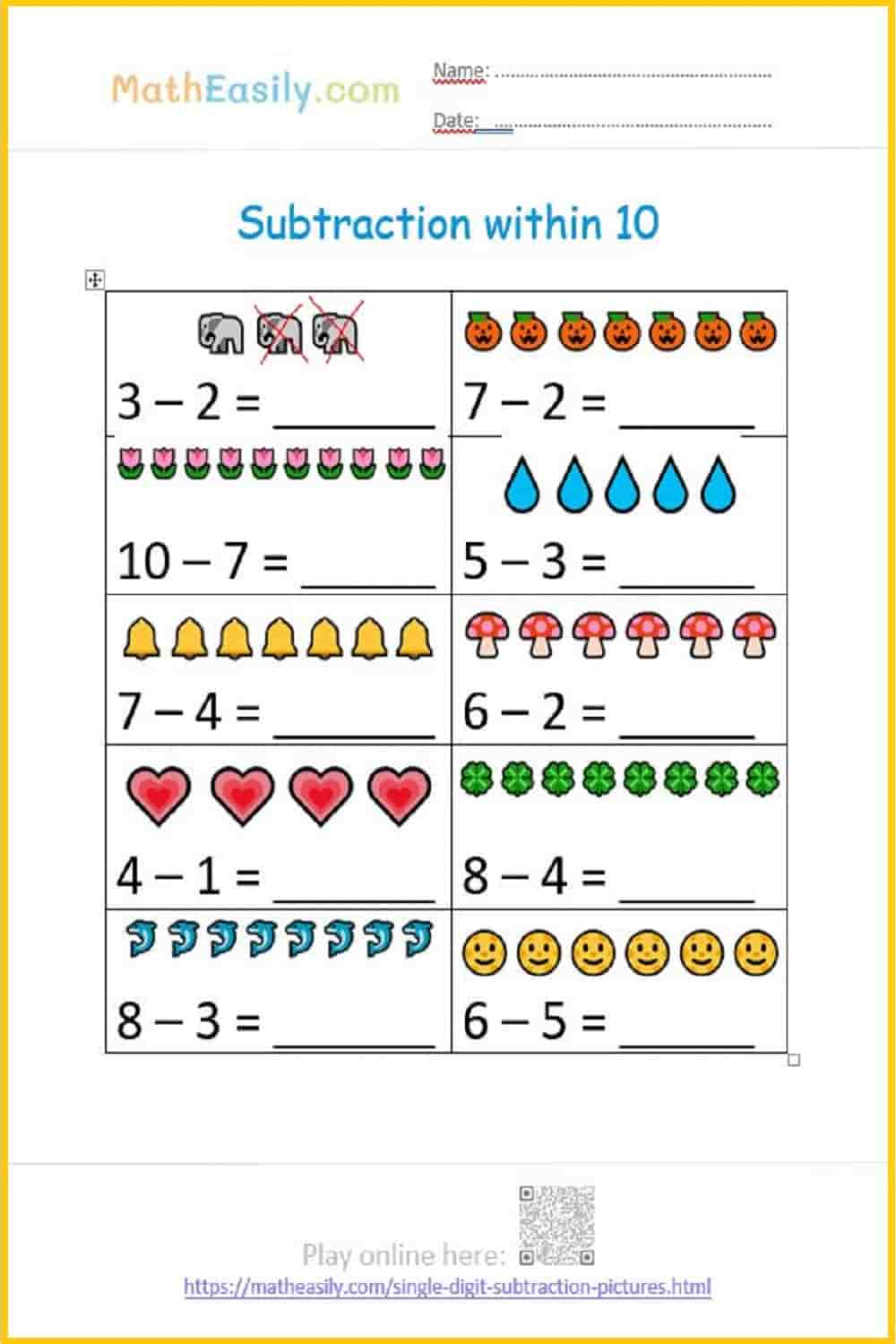### Subtraction up to 20

Subtraction within 20 with help of pictures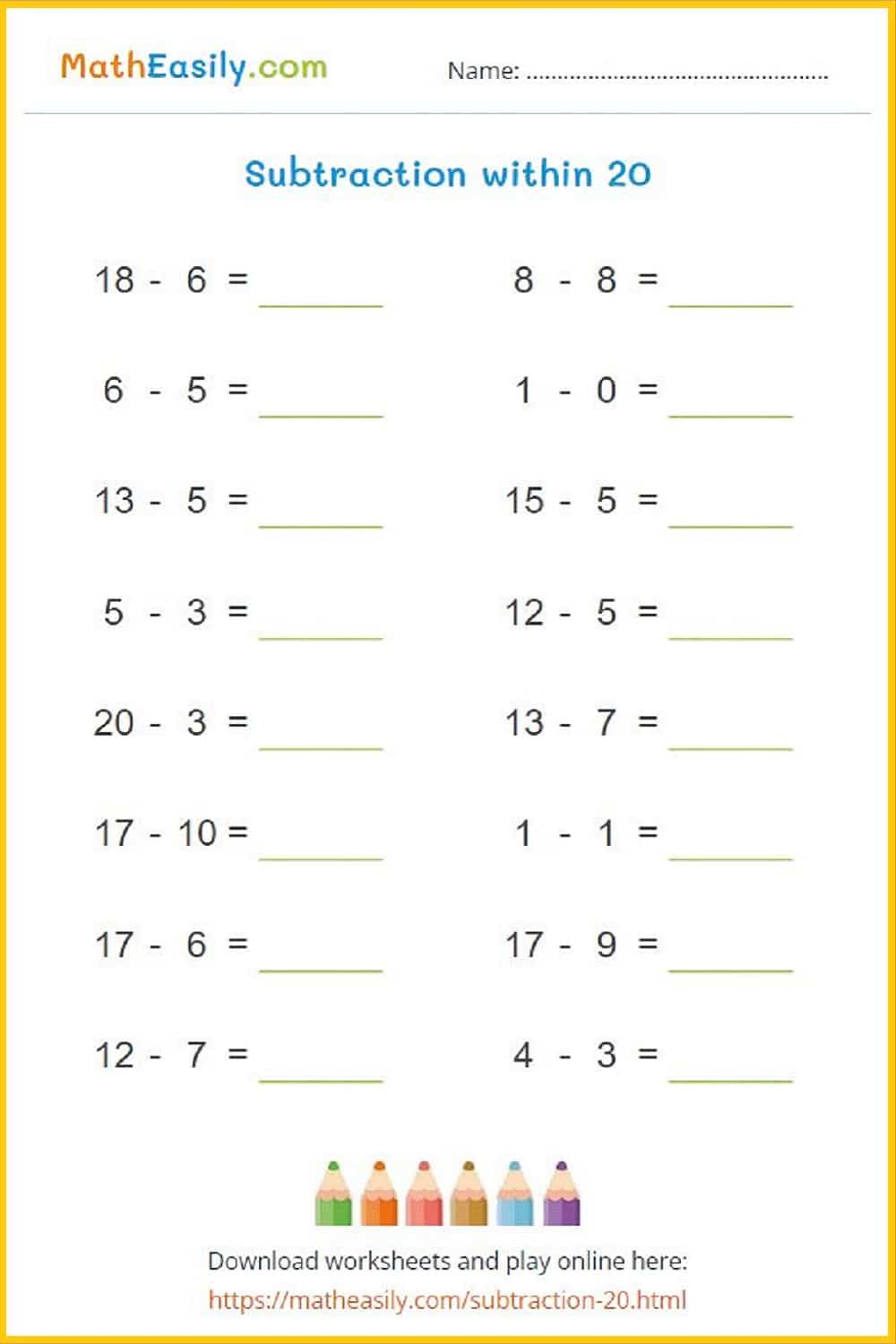### Subtraction up to 20

Subtraction within 20 with help of pictures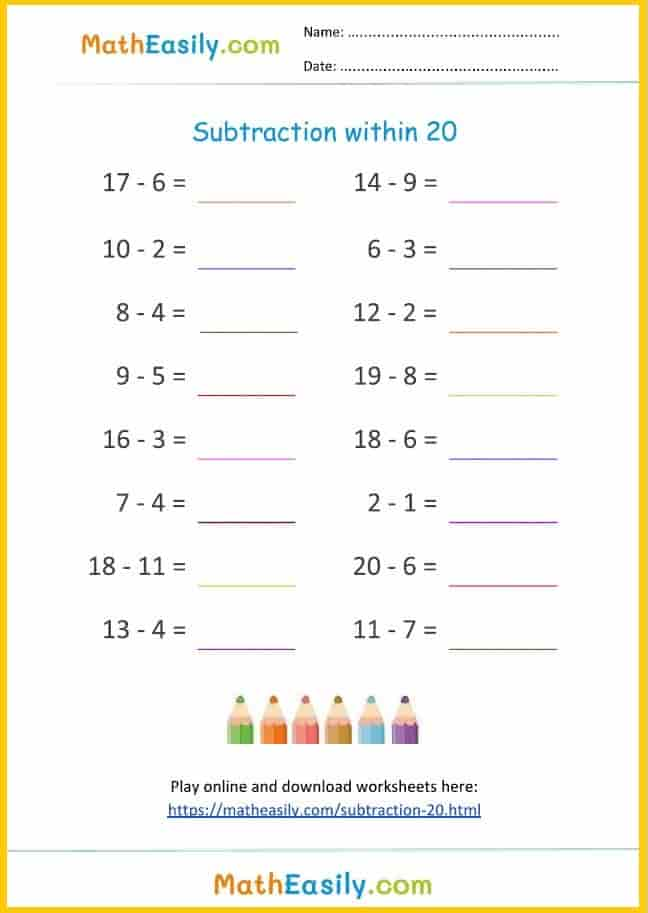### Subtraction pyramid

Fill in subtraction pyramid puzzles up to 30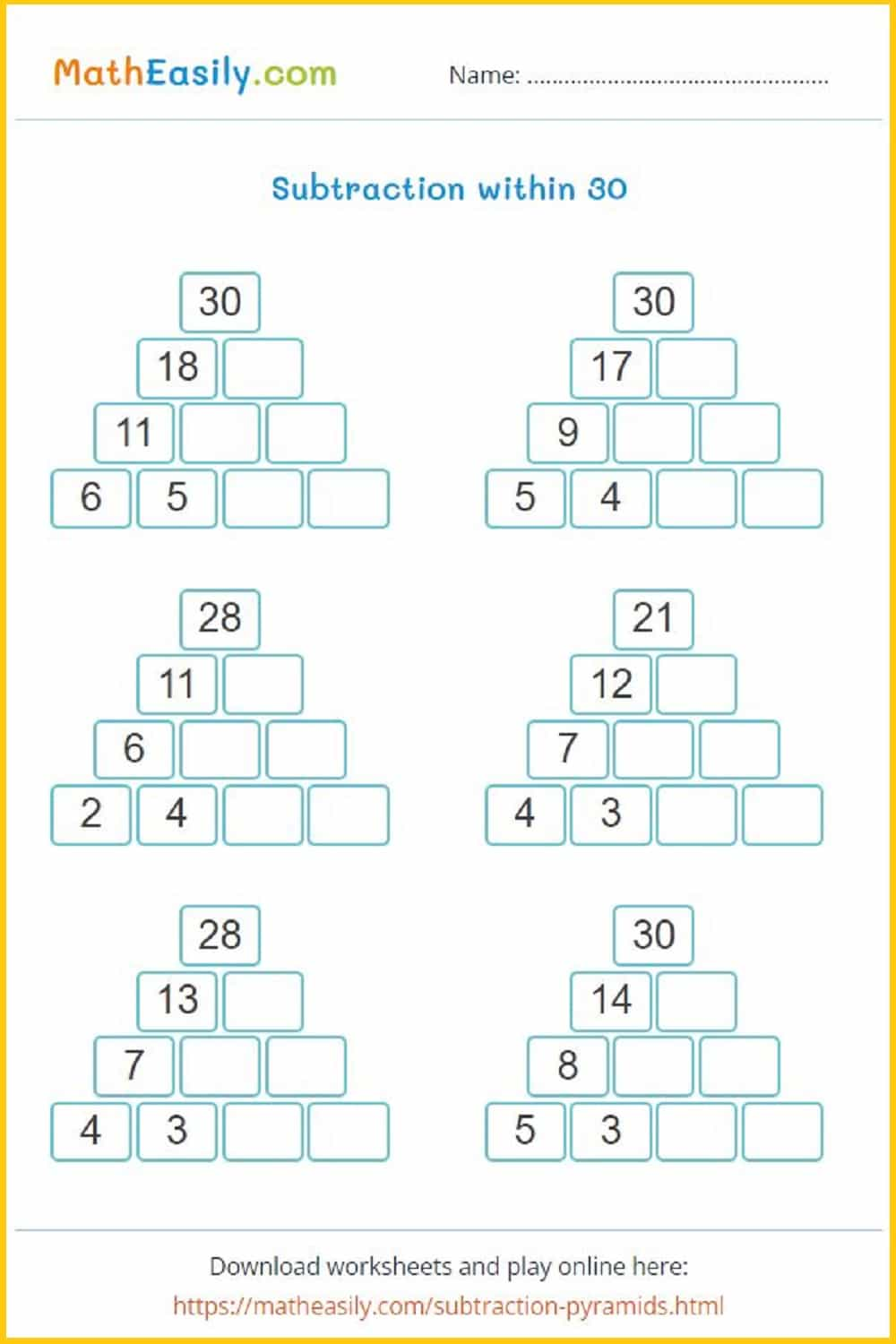## Multiplication for Kindergarten Worksheets PDF

Our printable multiplication worksheets for kindergarten help kids to learn basics of multiplication.

### Multiplication board game

Learning multiplication is one of the most challenging tasks in math. To help our kids to learn it easily I designed this printable multiplication board game in PDF.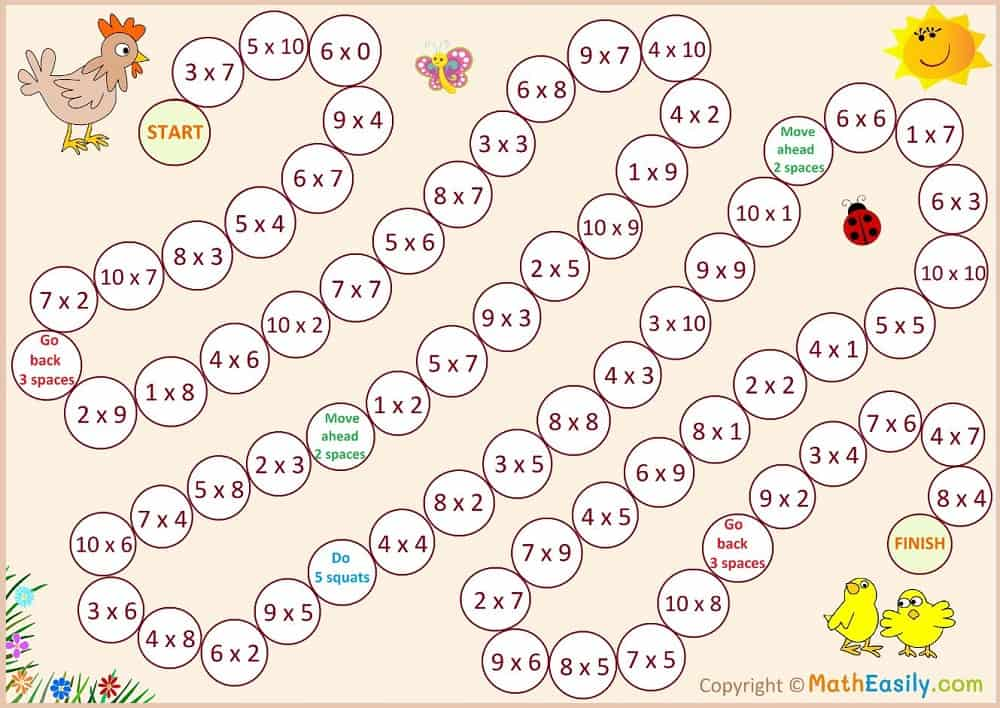### Multiplication table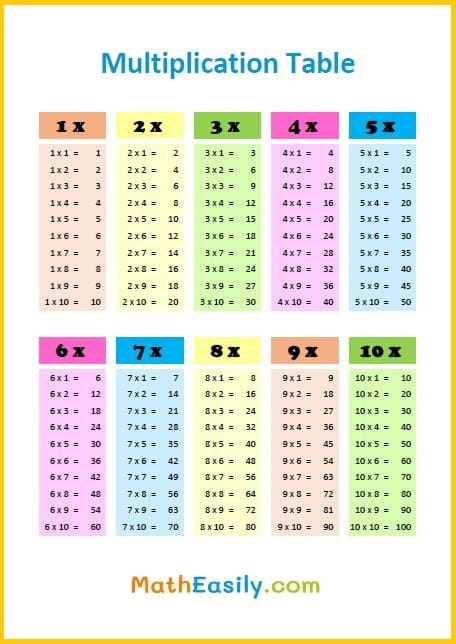### Multiplication dice game

Play easy multiplication game with dice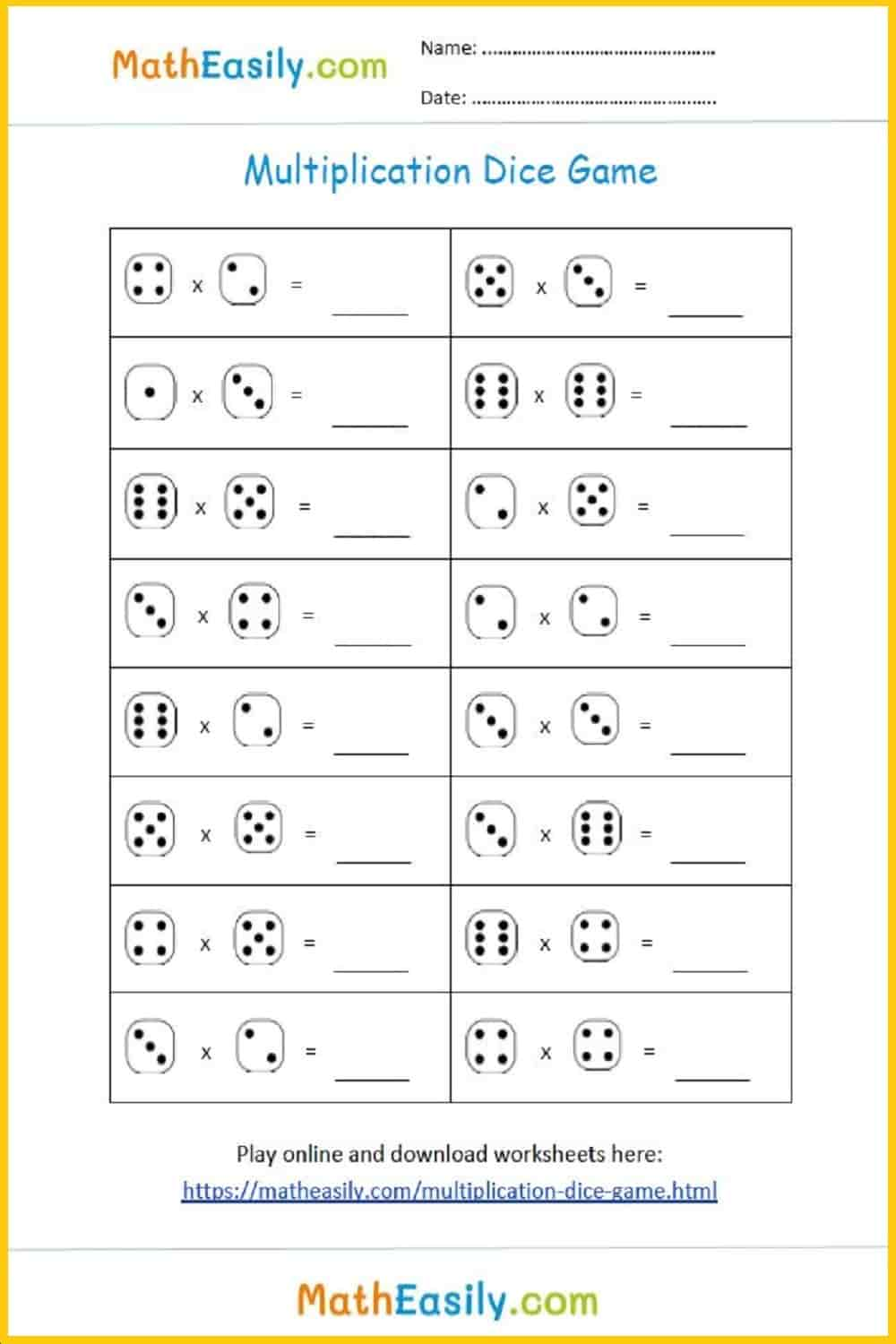### Multiplication dice game

Play easy multiplication game with dice### Single digit multiplication

Practice single digit multiplication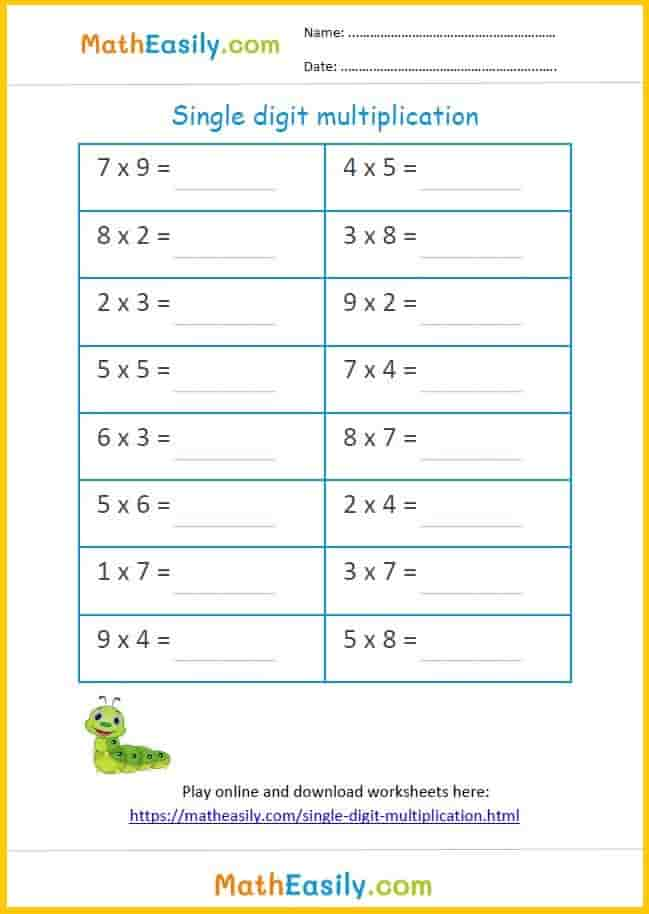### Single digit multiplication

Practice single digit multiplication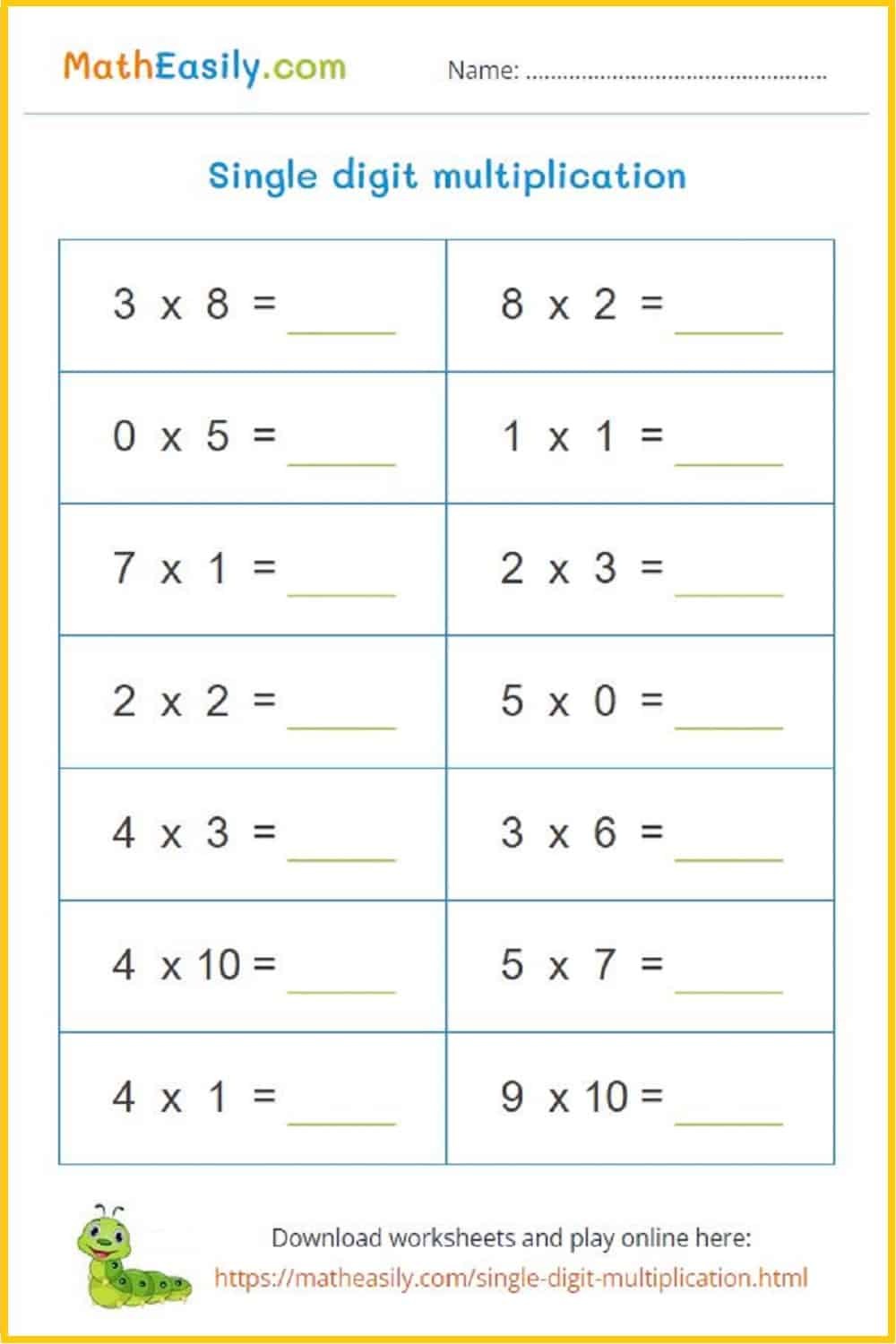## Common Core Kindergarten Math Worksheets Free Printable

Our common core kindergarten math worksheets are PDF documents which you can easily download. Just click on the image of kindergarten math worksheet and download the printable PDF. Each of these printable Kindergarten Math Worksheets contains also the link to the online game where kids can practice a given math skill.